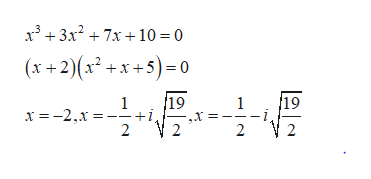# Find the SUM of all the complex zeros of f(x)= x3 + 3x2 + 7x + 10a.) -1  b.) -2  c.) -3  d.) 0  e.) 1

Question
2 views

Find the SUM of all the complex zeros of f(x)= x3 + 3x2 + 7x + 10

a.) -1  b.) -2  c.) -3  d.) 0  e.) 1

check_circle

Step 1

It is given that

Step 2

The roots of the given functi...help_outlineImage Transcriptionclosex³ + 3x? + 7x +10 = 0 (x +2)(x² +x +5)= 0 19 19 x =-2,x = +1 2 fullscreen

### Want to see the full answer?

See Solution

#### Want to see this answer and more?

Solutions are written by subject experts who are available 24/7. Questions are typically answered within 1 hour.*

See Solution
*Response times may vary by subject and question.
Tagged in

### Equations and In-equations